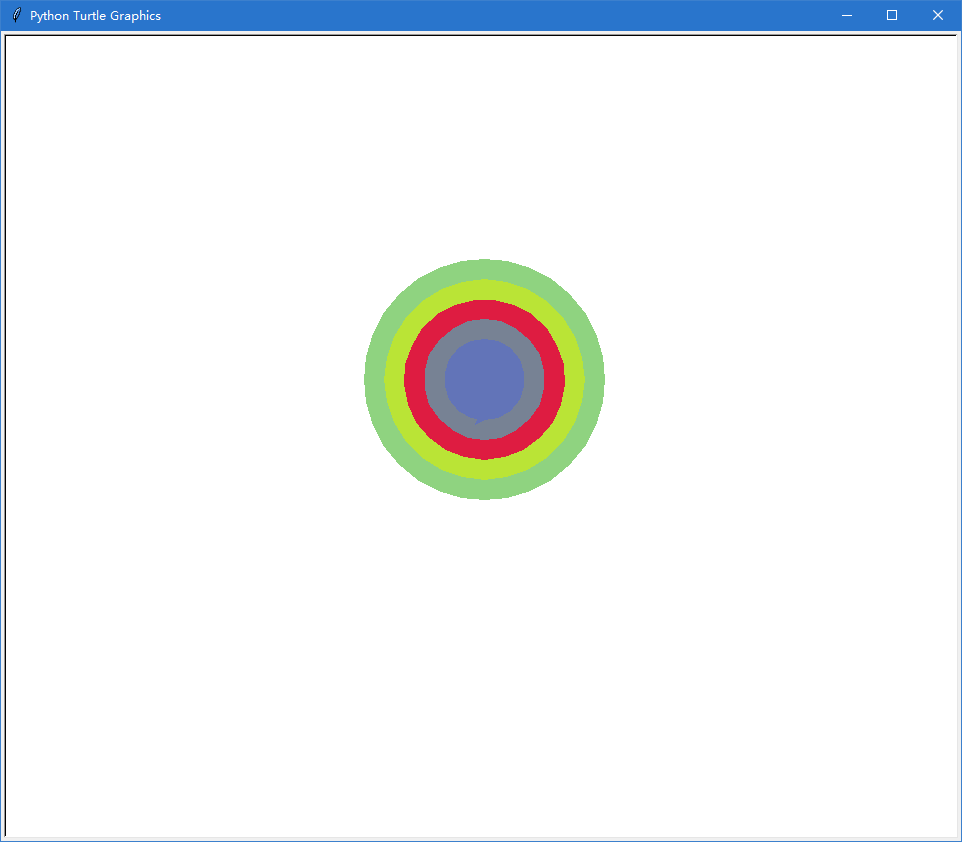2021-05-16 17:29

# 请问怎么样Python的turtle函数画随机填色的同心圆

• 写回答
• 好问题 提建议
• 关注问题
• 收藏
• 邀请回答

#### 3条回答默认 最新

•已采纳
``````import turtle
import random

for i in range(5):
color = "#{:0>6x}".format(random.randint(0x0,0xffffff))
print(color)
turtle.fillcolor(color)
turtle.pencolor(color)
turtle.up()
turtle.goto(0,(-60)+i*20)
turtle.seth(0)
turtle.down()
turtle.begin_fill()
turtle.circle(120-i*20)
turtle.end_fill()
turtle.done()
``````已采纳该答案
评论
解决 1 无用
打赏 举报
•``````import turtle
import random

#生成随机颜色（16进制）
def randomcolor():
colorArr = ['0','1','2','3','4','5','6','7','8','9','A','B','C','D','E','F']
color = ""
for i in range(6):
color += colorArr[random.randint(0,15)]
return "#"+color

t = turtle.Pen()
t.width(4)
t.speed(8)

for i in range(5):
t.penup()
t.goto(0,-50*i)
t.pendown()
r = random.randrange(0,257,10)
g = random.randrange(0,257,10)
b = random.randrange(0,257,10)
turtle.colormode(255)   # 需要将turtle.colormode设置为255以提供Hex代码(16进制)或R G B中的颜色字符串.
# t.color(r,g,b)                       # RGB颜色格式
t.color( randomcolor())         # HEX格式
t.circle(100+i * 50)
turtle.done()  # 程序执行完窗口仍然在``````
评论
解决 无用
打赏 举报
•有问必答小助手 2021-05-17 16:33

您好，我是有问必答小助手，您的问题已经有小伙伴解答了，您看下是否解决，可以追评进行沟通哦~

如果有您比较满意的答案 / 帮您提供解决思路的答案，可以点击【采纳】按钮，给回答的小伙伴一些鼓励哦～～